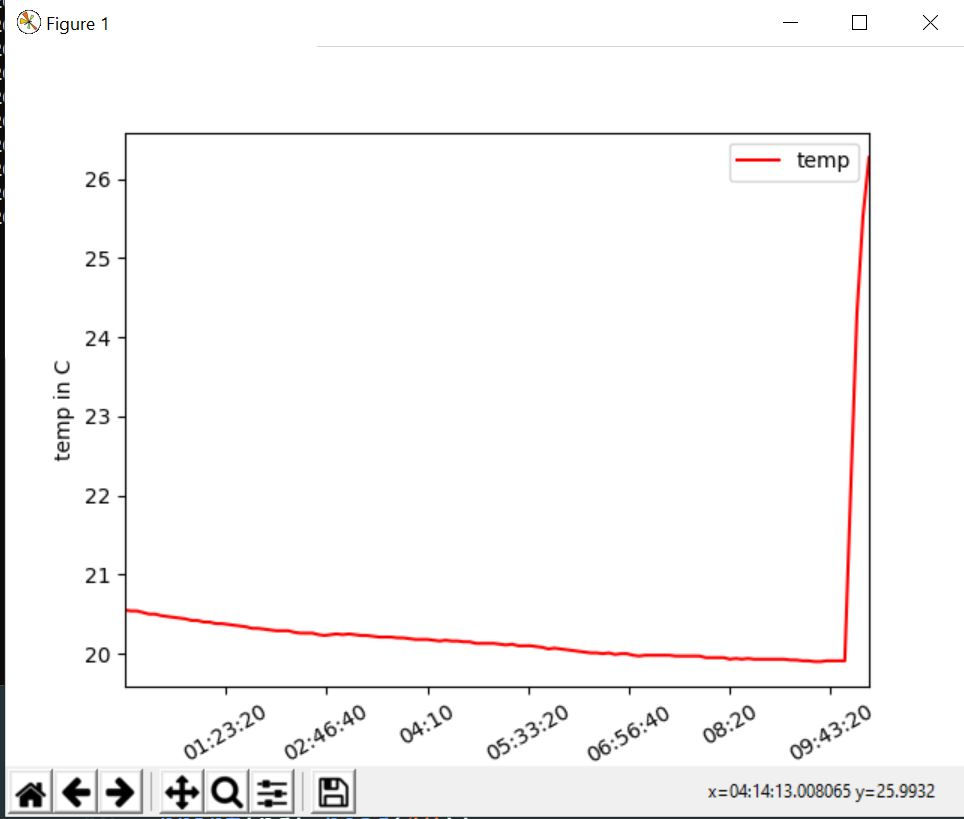# How to format Pandas / Matplotlib graph so the x-axis ticks are ONLY hours and minutes?

I am trying to plot time / temperature data from a csv file.

My goal is to have a graph which shows the temperature data per day.

My problem is the x-axis: i would like to show the time for uniformly and only be in Hours and minutes with 15 minute intervals, for example: 00:00, 00:15, 00:30

The csv is loaded into a pandas dataframe, where i filter the data to be shown based on what day it is, in the code i want only temperature data for 18th day of the month.

``````date,temp,humid
2020-10-17 23:50:02,20.57,87.5
2020-10-17 23:55:02,20.57,87.5
2020-10-18 00:00:02,20.55,87.31
2020-10-18 00:05:02,20.54,87.17
2020-10-18 00:10:02,20.54,87.16
2020-10-18 00:15:02,20.52,87.22
2020-10-18 00:20:02,20.5,87.24
2020-10-18 00:25:02,20.5,87.24
``````

here is the python code to make the graph:

``````import pandas as pd
import datetime
import matplotlib.pyplot as plt

#make new columns in dataframe so data can be filtered
df["New_Date"] = pd.to_datetime(df["date"]).dt.date
df["New_Time"] = pd.to_datetime(df["date"]).dt.time
df["New_hrs"] = pd.to_datetime(df["date"]).dt.hour
df["New_mins"] = pd.to_datetime(df["date"]).dt.minute
df["day"] = pd.DatetimeIndex(df['New_Date']).day

#filter the data to be only day 18
ndf = df[df["day"]==18]

#display dataframe in console
pd.set_option('display.max_rows', ndf.shape+1)

#plot a graph
ndf.plot(kind='line',x='New_Time',y='temp',color='red')

#edit graph to be sexy
plt.setp(plt.gca().xaxis.get_majorticklabels(),'rotation', 30)
plt.xlabel("time")
plt.ylabel("temp in C")

#show graph with the sexiness edits
plt.show()
``````

here is the graph i get: